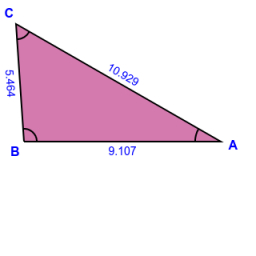# Circumference 71304

The PQR triangle with a circumference of 25.5 cm has sides in a ratio of 4: 6: 5. Determine the lengths of its sides.

p =  6.8 cm
q =  10.2 cm
r =  8.5 cm

### Step-by-step explanation:

Try calculation via our triangle calculator.Did you find an error or inaccuracy? Feel free to write us. Thank you!

Tips for related online calculators
Need help calculating sum, simplifying, or multiplying fractions? Try our fraction calculator.
Check out our ratio calculator.
Do you want to convert length units?
Do you want to convert time units like minutes to seconds?